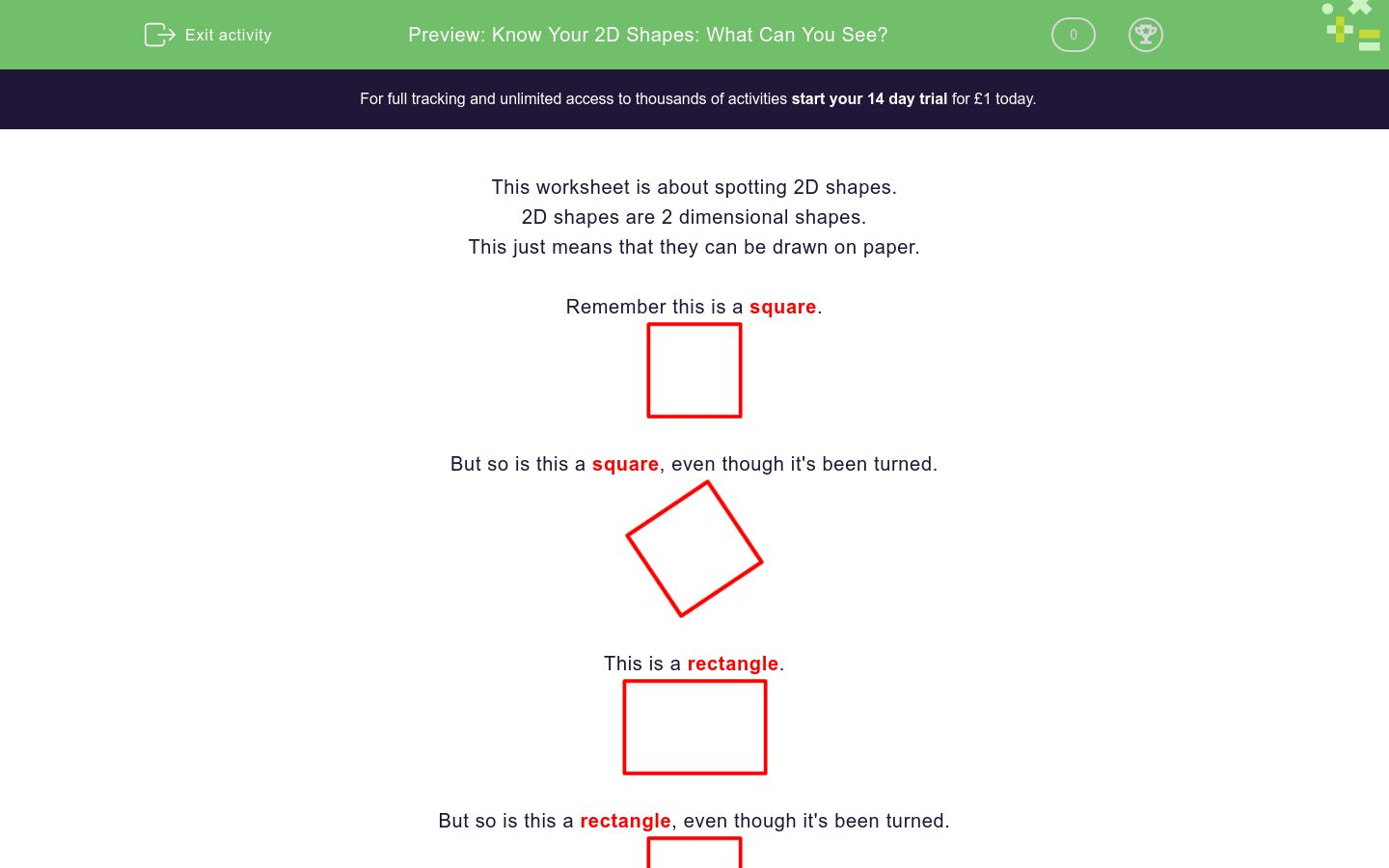# Know Your 2D Shapes: What Can You See?

In this worksheet, students look at 'disguised' 2D shapes and name them.Key stage:  KS 1

Curriculum topic:   Geometry: Properties of Shapes

Curriculum subtopic:   Recognise 2D and 3D Shapes

Difficulty level:### QUESTION 1 of 10

This worksheet is about spotting 2D shapes.

2D shapes are 2 dimensional shapes.

This just means that they can be drawn on paper.

Remember this is a square.But so is this a square, even though it's been turned.This is a rectangle.But so is this a rectangle, even though it's been turned.This is a triangle.This is a circle.Example

The picture is made from 2D shapes.

Which shapes can you see?There is...

• A yellow triangle
• A white circle
• A blue rectangle

The answer is a triangle, a circle and a rectangle.

The picture is made from 2D shapes.

Choose the names of the shapes you can see here.triangle

square

rectangle

circle

The picture is made from 2D shapes.

Choose the names of the shapes you can see here.triangle

square

rectangle

circle

The picture is made from 2D shapes.

Choose the names of the shapes you can see here.triangle

square

rectangle

circle

The picture is made from 2D shapes.

Choose the names of the shapes you can see here.triangle

square

rectangle

circle

The picture is made from 2D shapes.

Choose the names of the shapes you can see here.triangle

square

rectangle

circle

The picture is made from 2D shapes.

Choose the names of the shapes you can see here.triangle

square

rectangle

circle

The picture is made from 2D shapes.

Choose the names of the shapes you can see here.triangle

square

rectangle

circle

The picture is made from 2D shapes.

Choose the names of the shapes you can see here.triangle

square

rectangle

circle

The picture is made from 2D shapes.

Choose the names of the shapes you can see here.triangle

square

rectangle

circle

The picture is made from 2D shapes.

Choose the names of the shapes you can see here.

(Be careful!)triangle

square

rectangle

circle

• Question 1

The picture is made from 2D shapes.

Choose the names of the shapes you can see here.triangle
rectangle
EDDIE SAYS
A white triangle and a blue rectangle.
• Question 2

The picture is made from 2D shapes.

Choose the names of the shapes you can see here.rectangle
circle
EDDIE SAYS
A blue rectangle with a pink circle above it.
• Question 3

The picture is made from 2D shapes.

Choose the names of the shapes you can see here.triangle
circle
EDDIE SAYS
An orange triangle with a pink circle above it.
• Question 4

The picture is made from 2D shapes.

Choose the names of the shapes you can see here.triangle
square
EDDIE SAYS
A pink triangle with a blue square inside.
• Question 5

The picture is made from 2D shapes.

Choose the names of the shapes you can see here.rectangle
circle
EDDIE SAYS
A blue rectangle with 2 pink circles inside.
• Question 6

The picture is made from 2D shapes.

Choose the names of the shapes you can see here.triangle
rectangle
EDDIE SAYS
A white triangle with rectangles on each side.
• Question 7

The picture is made from 2D shapes.

Choose the names of the shapes you can see here.triangle
circle
EDDIE SAYS
3 black triangles and a small circle all inside a large circle.
• Question 8

The picture is made from 2D shapes.

Choose the names of the shapes you can see here.triangle
square
EDDIE SAYS
A blue square with pink triangles on each side.
• Question 9

The picture is made from 2D shapes.

Choose the names of the shapes you can see here.triangle
square
EDDIE SAYS
A blue square with a pink triangle inside.
• Question 10

The picture is made from 2D shapes.

Choose the names of the shapes you can see here.

(Be careful!)triangle
square
rectangle
EDDIE SAYS
A blue square with a pink triangle inside. There is also a blue rectangle above the pink triangle, and 2 blue triangles below the pink triangle too.
---- OR ----

Sign up for a £1 trial so you can track and measure your child's progress on this activity.

### What is EdPlace?

We're your National Curriculum aligned online education content provider helping each child succeed in English, maths and science from year 1 to GCSE. With an EdPlace account you’ll be able to track and measure progress, helping each child achieve their best. We build confidence and attainment by personalising each child’s learning at a level that suits them.

Get started